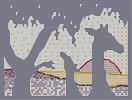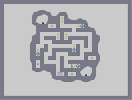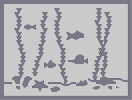### Unfinished White stripesHover over the thumbnail for a full-size version.

Author BE_nSPIRED author:be_nspired n-art people rated stripes unfinished white 2009-12-28 2009-12-28 4 by 15 people. \$Unfinished White stripes#BE_nSPIRED#white#00000000000000000000000000000000000000000000000000000000000000000000000000000000000000000000000000000000000000000000000000000000000000000000000000000000000000000000000000000000000000000000000000000000000000000000000000000000000000000000000000000000000000000000000000000000000000000000000000000000000000000000000000000000000000000000000000000000000000000000000000000000000000000000000000000000000000000000000000000000000000000000000000000000000000000000000000000000000000000000000000000000000000000000000000000000000000000000000000000000000000000000000000000000000000000000000000000000000000000000000000000000000000000000000000000000000000000000000000000000000000000000000000000000000000000000000000000000000000000|12^300,72!12^312,72!12^306,72!12^306,78!12^306,84!12^300,84!12^312,84!12^324,84!12^324,90!12^324,96!12^330,84!12^336,84!12^336,90!12^336,96!12^360,90!12^354,90!12^348,90!12^348,96!12^348,102!12^354,102!12^360,102!12^360,108!12^360,114!12^354,114!12^348,114!12^372,120!12^372,132!12^372,144!12^372,138!12^372,126!12^378,120!12^384,120!12^390,120!12^390,126!12^390,132!12^390,138!12^384,138!12^378,138!12^372,150!12^372,156!12^402,162!12^402,168!12^402,174!12^402,186!12^402,180!12^402,192!12^402,198!12^396,162!12^408,162!12^390,162!12^414,162!12^408,198!12^396,198!12^390,228!12^390,234!12^390,240!12^390,246!12^390,252!12^390,258!12^396,228!12^402,228!12^402,234!12^402,240!12^396,246!12^402,252!12^408,258!12^414,276!12^414,282!12^414,288!12^414,294!12^414,300!12^414,306!12^420,306!12^426,306!12^420,288!12^420,276!12^426,276!12^444,300!12^444,306!12^444,312!12^444,318!12^444,324!12^444,330!12^444,336!12^450,330!12^456,324!12^456,318!12^456,312!12^456,306!12^450,300!12^300,222!12^306,222!12^312,228!12^318,228!12^324,222!12^312,222!12^318,222!12^318,216!12^312,216!12^306,216!12^306,210!12^306,204!12^300,198!12^300,192!12^306,186!12^306,180!12^306,174!12^306,162!12^312,156!12^306,168!12^312,210!12^318,150!12^324,150!12^330,144!12^336,144!12^330,252!12^324,252!12^318,258!12^312,258!12^306,258!12^300,258!12^294,264!12^336,180!12^336,174!12^318,174!12^330,168!12^354,174!12^354,168!12^360,168!12^360,174!12^366,180!12^360,180!12^354,180!12^348,180!12^234,138!12^234,144!12^234,150!12^234,156!12^234,162!12^234,168!12^234,174!12^234,180!12^234,186!12^234,198!12^234,192!12^240,204!12^240,210!12^240,222!12^234,216!12^234,228!12^240,216!12^234,222!12^228,234!12^234,210!12^228,228!12^228,216!12^228,222!12^228,210!12^228,204!12^234,204!12^228,198!12^228,192!12^228,186!12^228,180!12^228,174!12^228,168!12^228,162!12^228,156!12^228,150!12^234,138!12^228,138!12^228,144!12^228,240!12^228,246!12^234,252!12^234,258!12^234,264!12^240,270!12^246,282!12^252,282!12^240,276!12^258,288!12^246,276!12^240,264!12^240,132!12^240,126!12^246,120!12^246,114!12^252,108!12^258,102!12^264,96!12^270,90!12^276,90!12^222,222!12^222,216!12^222,210!12^222,204!12^222,198!12^222,192!12^222,186!12^222,180!12^222,174!12^222,168!12^222,162!12^222,156!12^222,150!12^222,144!12^222,138!12^228,132!12^234,132!12^222,132!12^228,126!12^234,126!12^234,120!12^240,120!12^240,114!12^234,114!12^240,108!12^246,108!12^246,102!12^252,102!12^252,96!12^258,96!12^258,90!12^264,90!12^276,72!12^276,84!12^264,84!12^270,78!12^270,84!12^264,78!12^258,84!12^246,90!12^252,90!12^246,96!12^234,102!12^240,102!12^240,96!12^222,126!12^222,120!12^228,120!12^228,114!12^234,102!12^228,108!12^234,108!12^216,132!12^216,144!12^216,138!12^216,150!12^216,156!12^216,162!12^216,174!12^216,198!12^216,192!12^216,186!12^216,180!12^216,168!12^210,186!12^210,180!12^210,168!12^210,162!12^210,174!12^210,192!12^276,78!12^282,78!12^288,78!12^294,78!12^306,78!12^312,78!12^300,78!12^300,72!12^288,72!12^282,72!12^294,72!12^294,66!12^300,66!12^312,66!12^318,66!12^330,66!12^336,66!12^348,66!12^324,66!12^342,66!12^306,66!12^288,66!12^318,72!12^324,72!12^330,72!12^336,72!12^342,72!12^342,72!12^348,72!12^360,72!12^354,72!12^366,72!12^372,72!12^378,72!12^390,72!12^384,72!12^288,84!12^282,84!12^294,84!12^300,60!12^306,60!12^312,60!12^318,60!12^324,60!12^330,60!12^378,78!12^384,78!12^390,78!12^396,78!12^402,78!12^402,84!12^396,84!12^384,84!12^384,84!12^384,84!12^390,84!12^354,66!12^360,66!12^408,84!12^414,90!12^420,90!12^420,96!12^426,96!12^408,96!12^414,96!12^408,90!12^402,90!12^396,90!12^318,78!12^324,78!12^330,78!12^336,78!12^342,78!12^348,78!12^354,78!12^366,78!12^318,84!12^318,96!12^312,90!12^318,90!12^306,90!12^312,96!12^324,108!12^324,102!12^318,102!12^330,90!12^330,96!12^330,102!12^330,114!12^330,108!12^330,120!12^330,126!12^336,126!12^336,120!12^336,114!12^336,108!12^336,102!12^342,102!12^342,96!12^342,90!12^342,84!12^348,84!12^354,84!12^360,84!12^360,78!12^366,84!12^372,84!12^372,78!12^378,96!12^384,84!12^378,84!12^378,90!12^372,90!12^366,90!12^366,96!12^360,96!12^354,96!12^354,108!12^348,108!12^342,108!12^342,114!12^438,150!12^444,150!12^450,150!12^456,156!12^456,150!12^462,150!12^468,150!12^474,150!12^480,150!12^486,150!12^492,150!12^492,144!12^486,144!12^480,144!12^480,144!12^474,144!12^468,144!12^462,144!12^456,144!12^450,144!12^444,144!12^444,144!12^438,144!12^438,144!12^438,144!12^432,144!12^426,144!12^426,144!12^420,144!12^420,138!12^420,132!12^420,132!12^420,126!12^420,120!12^420,114!12^420,108!12^420,102!12^342,120!12^348,120!12^354,120!12^342,126!12^348,126!12^354,126!12^360,126!12^360,126!12^366,126!12^366,120!12^360,120!12^366,114!12^372,114!12^366,108!12^372,108!12^372,102!12^372,96!12^366,102!12^378,102!12^384,102!12^384,90!12^384,96!12^390,90!12^396,96!12^390,96!12^402,96!12^402,102!12^408,102!12^414,102!12^414,108!12^402,108!12^402,108!12^408,108!12^396,108!12^390,108!12^384,108!12^378,108!12^252,204!12^252,198!12^348,174!12^342,174!12^342,168!12^348,162!12^348,168!12^354,162!12^360,162!12^360,156!12^354,156!12^354,150!12^348,144!12^342,144!12^342,138!12^348,138!12^354,138!12^354,144!12^360,144!12^360,150!12^360,156!12^366,156!12^366,150!12^366,144!12^366,138!12^366,132!12^360,132!12^354,132!12^348,132!12^342,132!12^336,132!12^324,114!12^318,108!12^360,138!12^390,102!12^396,102!12^378,114!12^384,114!12^390,114!12^396,114!12^402,114!12^408,114!12^414,114!12^414,120!12^408,120!12^402,120!12^396,120!12^378,126!12^384,126!12^384,132!12^378,132!12^396,126!12^402,126!12^408,126!12^408,126!12^414,126!12^252,174!12^246,174!12^426,138!12^432,138!12^438,138!12^444,138!12^450,138!12^456,138!12^462,138!12^468,138!12^474,138!12^480,138!12^486,138!12^492,138!12^492,132!12^486,132!12^474,138!12^474,132!12^480,132!12^468,132!12^462,132!12^456,132!12^450,132!12^444,132!12^438,132!12^432,132!12^426,132!12^426,126!12^426,120!12^426,114!12^426,108!12^426,96!12^432,102!12^426,102!12^432,108!12^432,114!12^432,120!12^432,126!12^438,126!12^444,126!12^450,126!12^456,126!12^462,126!12^456,120!12^444,120!12^450,120!12^438,120!12^438,114!12^438,108!12^414,150!12^420,150!12^426,150!12^432,150!12^426,156!12^420,156!12^414,156!12^408,156!12^408,150!12^408,144!12^414,144!12^414,138!12^414,132!12^408,132!12^402,132!12^396,132!12^402,138!12^408,138!12^396,138!12^396,144!12^402,144!12^402,150!12^402,156!12^396,156!12^396,150!12^384,156!12^390,150!12^390,156!12^390,144!12^384,144!12^378,144!12^378,150!12^384,150!12^378,156!12^366,162!12^372,162!12^378,162!12^384,162!12^384,174!12^384,174!12^384,168!12^390,168!12^396,168!12^396,174!12^390,174!12^396,180!12^384,180!12^390,180!12^378,180!12^372,180!12^372,174!12^366,174!12^366,168!12^372,168!12^378,168!12^378,174!12^450,156!12^444,156!12^438,156!12^432,156!12^432,168!12^432,162!12^432,174!12^432,180!12^432,186!12^432,192!12^432,198!12^432,204!12^432,210!12^432,216!12^432,222!12^432,234!12^432,228!12^282,90!12^276,96!12^270,96!12^264,102!12^258,108!12^252,114!12^258,114!12^252,120!12^246,126!12^246,132!12^246,138!12^246,144!12^246,156!12^246,150!12^246,162!12^246,168!12^240,168!12^240,162!12^240,156!12^240,150!12^240,144!12^240,138!12^240,174!12^240,180!12^240,186!12^240,192!12^240,198!12^246,204!12^246,198!12^246,186!12^246,192!12^258,174!12^264,174!12^240,252!12^240,246!12^234,246!12^234,240!12^240,240!12^240,234!12^234,234!12^240,228!12^252,216!12^246,216!12^246,210!12^252,210!12^246,222!12^252,276!12^252,270!12^252,264!12^246,258!12^246,252!12^366,186!12^372,186!12^378,186!12^384,186!12^390,186!12^396,186!12^396,192!12^390,192!12^384,192!12^372,192!12^378,192!12^264,198!12^228,252!12^228,258!12^228,264!12^228,270!12^264,294!12^378,198!12^378,204!12^378,210!12^378,210!12^378,216!12^378,222!12^378,228!12^378,234!12^378,240!12^378,252!12^378,246!12^372,252!12^366,258!12^366,270!12^384,198!12^384,204!12^384,210!12^384,216!12^384,222!12^384,228!12^384,234!12^384,240!12^384,246!12^384,252!12^390,216!12^390,222!12^390,210!12^390,204!12^390,198!12^396,204!12^396,204!12^396,210!12^396,222!12^396,216!12^396,234!12^396,240!12^408,234!12^408,228!12^408,222!12^402,222!12^402,216!12^402,210!12^402,204!12^408,204!12^408,210!12^408,216!12^414,216!12^414,210!12^414,204!12^414,198!12^414,192!12^408,192!12^408,186!12^408,180!12^408,174!12^408,168!12^414,168!12^414,174!12^414,180!12^414,186!12^420,186!12^420,174!12^420,174!12^420,162!12^420,168!12^426,162!12^426,174!12^426,168!12^426,186!12^420,180!12^426,180!12^420,198!12^420,192!12^426,192!12^426,198!12^420,204!12^426,204!12^426,216!12^420,210!12^426,210!12^426,222!12^420,222!12^420,216!12^414,222!12^414,228!12^420,228!12^426,228!12^426,234!12^420,234!12^414,234!12^414,240!12^408,240!12^408,246!12^402,246!12^408,252!12^414,252!12^414,252!12^414,246!12^420,246!12^420,240!12^426,240!12^432,240!12^432,246!12^426,246!12^426,252!12^420,252!12^420,258!12^414,258!12^414,264!12^408,264!12^402,264!12^402,258!12^396,258!12^396,252!12^222,222!12^222,228!12^438,162!12^444,162!12^450,162!12^450,162!12^408,270!12^408,276!12^408,282!12^408,288!12^402,288!12^402,282!12^402,276!12^402,270!12^396,264!12^396,270!12^396,270!12^396,276!12^396,282!12^396,288!12^390,288!12^390,282!12^390,276!12^390,270!12^390,264!12^384,264!12^384,258!12^378,258!12^372,258!12^372,264!12^366,264!12^378,264!12^378,270!12^384,270!12^372,270!12^366,276!12^372,276!12^378,276!12^384,276!12^384,282!12^378,282!12^372,282!12^372,282!12^366,282!12^366,288!12^372,288!12^378,288!12^384,288!12^354,294!12^360,294!12^366,294!12^372,294!12^384,294!12^378,294!12^390,294!12^396,294!12^408,294!12^348,300!12^354,300!12^360,300!12^366,300!12^372,300!12^378,300!12^384,300!12^291,88!12^291,90!12^288,93!12^285,94!12^283,96!12^281,97!12^278,98!12^274,100!12^272,101!12^272,102!12^268,105!12^265,107!12^265,109!12^265,113!12^258,116!12^258,118!12^258,119!12^250,125!12^250,128!12^250,131!12^247,139!12^258,123!12^258,123!12^256,126!12^254,129!12^252,132!12^252,133!12^250,137!12^246,160!12^249,170!12^249,171!12^251,175!12^253,175!12^255,175!12^257,173!12^259,173!12^261,173!12^262,173!12^263,174!12^243,175!12^243,180!12^246,182!12^243,228!12^243,231!12^243,234!12^243,239!12^244,244!12^244,247!12^244,255!12^231,258!12^234,260!12^238,262!12^238,266!12^244,272!12^246,275!12^247,262!12^251,221!12^268,196!12^270,196!12^273,195!12^273,185!12^267,185!12^262,185!12^257,187!12^255,189!12^253,191!12^249,193!12^321,170!12^326,167!12^335,167!12^330,177!12^347,184!12^344,187!12^342,188!12^300,88!12^300,91!12^304,95!12^306,98!12^307,100!12^308,102!12^309,104!12^312,107!12^313,110!12^314,113!12^316,117!12^318,122!12^324,124!12^327,124!12^330,128!12^332,131!12^334,136!12^338,139!12^340,140!12^325,119!12^325,119!12^323,117!12^323,114!12^316,109!12^316,103!12^312,100!12^309,96!12^320,118!12^286,89!12^294,219!12^296,219!12^297,215!12^299,215!12^300,213!12^300,211!12^302,208!12^303,203!12^304,189!12^304,192!12^304,196!12^304,201!12^303,182!12^303,182!12^303,180!12^303,179!12^299,177!12^299,174!12^300,173!12^301,171!12^302,169!12^303,168!12^308,155!12^308,167!12^309,164!12^311,158!12^313,154!12^315,150!12^319,146!12^323,146!12^328,147!12^330,147!12^330,147!12^334,147!12^341,147!12^347,148!12^352,151!12^322,253!12^322,253!12^317,254!12^314,257!12^312,257!12^310,257!12^310,255!12^306,255!12^305,255!12^304,255!12^303,256!12^301,258!12^332,254!12^299,262!12^357,182!12^358,183!12^361,187!12^363,190!12^365,192!12^367,195!12^369,197!12^369,199!12^373,203!12^373,205!12^373,207!12^373,196!12^371,252!12^371,250!12^372,247!12^374,245!12^374,244!12^374,241!12^374,239!12^374,237!12^376,233!12^376,231!12^363,285!12^363,285!12^359,288!12^355,291!12^352,295!12^350,297!12^348,301!12^343,304!12^341,305!12^339,308!12^336,311!12^333,313!12^330,314!12^324,316!12^321,316!12^317,316!12^309,318!12^342,306!12^348,306!12^348,306!12^354,306!12^354,306!12^360,306!12^366,306!12^366,306!12^372,306!12^378,306!12^384,312!12^384,306!12^390,300!12^390,306!12^396,306!12^396,300!12^402,300!12^402,294!12^402,306!12^408,306!12^408,300!12^342,312!12^348,312!12^348,312!12^354,312!12^360,312!12^366,312!12^372,312!12^378,312!12^378,318!12^372,318!12^366,318!12^366,318!12^360,318!12^354,318!12^348,318!12^342,318!12^330,318!12^336,318!12^324,324!12^312,324!12^318,324!12^330,324!12^336,324!12^342,324!12^348,324!12^354,324!12^360,324!12^366,324!12^372,324!12^378,324!12^324,318!12^318,318!12^253,274!12^254,278!12^255,281!12^257,285!12^259,287!12^261,290!12^263,292!12^265,295!12^265,298!12^267,299!12^269,300!12^271,302!12^271,304!12^272,307!12^275,310!12^277,311!12^279,312!12^228,272!12^228,274!12^231,280!12^231,282!12^232,285!12^233,287!12^233,289!12^233,294!12^254,293!12^254,294!12^254,298!12^254,301!12^254,304!12^254,309!12^258,297!12^260,302!12^263,310!12^266,312!12^267,315!12^268,318!12^263,306!12^254,290!12^252,288!12^252,286!12^252,285!12^219,231!12^220,237!12^220,240!12^220,243!12^221,250!12^223,253!12^222,248!12^222,239!12^222,235!12^222,230!12^218,224!12^218,220!12^218,216!12^218,212!12^216,206!12^214,201!12^214,197!12^209,193!12^207,195!12^207,200!12^207,204!12^207,207!12^207,212!12^208,215!12^209,217!12^210,219!12^210,221!12^210,222!12^210,224!12^209,169!12^207,171!12^207,172!12^204,175!12^201,176!12^199,176!12^198,177!12^195,178!12^209,155!12^210,151!12^210,145!12^212,136!12^212,133!12^215,129!12^218,122!12^221,117!12^225,110!12^228,101!12^231,99!12^237,94!12^212,134!12^204,128!12^204,125!12^204,121!12^208,115!12^210,113!12^210,111!12^214,106!12^217,101!12^218,97!12^221,93!12^223,90!12^226,88!12^229,86!12^234,86!12^238,84!12^241,82!12^243,82!12^247,80!12^253,80!12^256,80!12^257,80!12^257,82!12^254,86!12^251,87!12^249,90!12^245,93!12^239,97!12^235,99!12^230,103!12^228,107!12^223,114!12^220,123!12^217,129!12^263,74!12^265,72!12^269,70!12^271,70!12^275,66!12^294,59!12^291,61!12^288,62!12^284,64!12^284,66!12^281,66!12^277,68!12^334,60!12^335,60!12^337,60!12^341,61!12^343,61!12^350,61!12^354,62!12^364,64!12^367,65!12^371,67!12^375,68!12^377,68!12^382,69!12^388,71!12^409,80!12^411,82!12^413,83!12^416,85!12^417,86!12^418,88!12^439,102!12^439,104!12^443,106!12^445,106!12^446,106!12^433,102!12^433,99!12^431,96!12^426,94!12^395,74!12^398,74!12^286,223!12^286,223!12^284,226!12^284,227!12^286,227!12^308,225!12^321,227!12^312,321!12^324,330!12^330,330!12^336,330!12^342,330!12^348,330!12^354,330!12^360,330!12^366,330!12^378,330!12^372,330!12^378,342!12^372,342!12^372,336!12^378,336!12^366,336!12^360,336!12^354,336!12^348,336!12^342,336!12^336,336!12^330,336!12^390,312!12^402,312!12^396,312!12^384,318!12^348,342!12^354,342!12^360,342!12^366,342!12^366,348!12^360,348!12^372,348!12^378,348!12^384,348!12^396,348!12^390,348!12^384,354!12^372,354!12^378,354!12^366,354!12^360,354!12^414,270!12^420,270!12^420,264!12^426,264!12^426,258!12^432,252!12^432,258!12^432,264!12^432,270!12^426,270!12^420,282!12^426,282!12^426,288!12^426,294!12^420,294!12^420,300!12^426,300!12^432,300!12^432,294!12^432,288!12^432,282!12^432,276!12^438,276!12^438,270!12^438,264!12^438,258!12^438,252!12^438,246!12^462,156!12^474,156!12^468,156!12^480,156!12^486,156!12^468,174!12^474,174!12^480,174!12^486,174!12^492,174!12^498,174!12^504,174!12^504,168!12^498,168!12^492,168!12^480,168!12^480,168!12^486,168!12^474,168!12^468,168!12^462,174!12^456,168!12^468,168!12^462,168!12^450,168!12^438,168!12^438,174!12^444,168!12^444,174!12^450,174!12^456,174!12^450,270!12^450,270!12^450,276!12^450,282!12^450,282!12^450,288!12^456,288!12^462,288!12^462,288!12^468,288!12^468,288!12^468,288!12^474,288!12^468,282!12^462,282!12^456,282!12^456,276!12^462,276!12^468,276!12^468,270!12^462,270!12^456,270!12^456,264!12^450,264!12^450,258!12^444,258!12^444,252!12^444,246!12^438,240!12^438,240!12^438,228!12^438,234!12^438,222!12^438,216!12^438,210!12^438,204!12^438,192!12^438,192!12^438,198!12^438,186!12^438,180!12^444,222!12^486,180!12^498,180!12^492,180!12^498,186!12^510,186!12^504,186!12^504,180!12^510,180!12^510,174!12^510,168!12^510,162!12^504,162!12^498,162!12^492,162!12^486,162!12^474,162!12^480,162!12^462,162!12^468,162!12^456,162!12^426,312!12^426,318!12^426,324!12^426,330!12^432,330!12^432,324!12^432,318!12^432,312!12^432,306!12^438,330!12^438,324!12^438,318!12^438,312!12^438,306!12^438,300!12^438,294!12^438,282!12^438,288!12^444,288!12^444,282!12^444,276!12^444,270!12^444,258!12^444,264!12^516,192!12^522,186!12^516,186!12^516,180!12^522,180!12^522,174!12^516,174!12^516,168!12^516,162!12^516,156!12^510,156!12^504,156!12^498,156!12^492,156!12^420,312!12^420,318!12^420,324!12^420,330!12^414,330!12^414,324!12^414,318!12^414,312!12^408,312!12^444,294!12^456,294!12^450,294!12^450,312!12^450,306!12^450,318!12^450,324!12^456,300!12^462,300!12^462,294!12^468,294!12^474,294!12^480,294!12^498,150!12^498,144!12^498,138!12^498,132!12^498,126!12^492,126!12^486,126!12^480,126!12^474,126!12^468,126!12^462,120!12^468,120!12^474,120!12^480,120!12^486,120!12^498,120!12^492,120!12^504,150!12^504,144!12^504,138!12^504,132!12^504,126!12^462,306!12^468,306!12^468,300!12^468,312!12^462,318!12^462,312!12^468,318!12^468,324!12^462,324!12^462,330!12^462,336!12^462,342!12^462,348!12^462,354!12^462,360!12^462,372!12^462,366!12^462,378!12^462,384!12^462,390!12^462,396!12^456,396!12^456,396!12^456,390!12^456,384!12^456,378!12^456,372!12^456,366!12^456,354!12^456,336!12^456,330!12^456,342!12^456,348!12^450,336!12^450,348!12^450,342!12^456,360!12^450,360!12^450,354!12^450,366!12^450,378!12^450,372!12^450,384!12^450,390!12^450,402!12^450,396!12^510,144!12^510,150!12^510,138!12^510,132!12^510,126!12^516,132!12^516,138!12^516,144!12^516,150!12^522,138!12^522,144!12^522,150!12^522,156!12^522,162!12^522,168!12^528,144!12^528,150!12^528,156!12^528,162!12^528,168!12^528,174!12^528,180!12^528,192!12^528,186!12^522,192!12^522,198!12^468,234!12^462,234!12^474,228!12^480,228!12^522,216!12^534,216!12^528,216!12^528,204!12^528,210!12^534,210!12^534,198!12^528,198!12^534,204!12^534,192!12^534,186!12^534,180!12^534,174!12^534,168!12^534,162!12^534,156!12^534,150!12^373,212!12^373,216!12^374,217!12^374,221!12^374,225!12^441,113!12^443,116!12^447,117!12^453,117!12^459,117!12^461,117!12^463,117!12^468,117!12^472,117!12^475,117!12^477,115!12^484,115!12^486,115!12^489,115!12^495,115!12^497,115!12^500,117!12^503,120!12^505,120!12^508,123!12^515,127!12^516,128!12^520,132!12^522,133!12^524,137!12^526,140!12^528,141!12^530,144!12^530,147!12^313,326!12^313,326!12^315,328!12^317,331!12^319,332!12^324,334!12^327,336!12^329,338!12^333,339!12^339,341!12^342,342!12^344,343!12^347,345!12^350,347!12^354,348!12^356,350!12^358,356!12^367,360!12^368,360!12^373,360!12^362,360!12^382,345!12^382,345!12^382,345!12^386,322!12^387,320!12^392,318!12^395,319!12^399,322!12^401,324!12^403,327!12^407,330!12^409,332!12^411,332!12^409,322!12^406,318!12^403,318!12^396,316!12^405,322!12^407,322!12^409,316!12^388,318!12^450,175!12^450,177!12^447,179!12^442,181!12^440,183!12^440,188!12^440,193!12^440,199!12^440,201!12^440,208!12^440,213!12^440,216!12^441,219!12^442,222!12^443,222!12^445,223!12^443,226!12^443,228!12^443,232!12^443,237!12^443,240!12^444,244!12^446,249!12^450,252!12^452,256!12^454,260!12^456,264!12^460,266!12^462,266!12^465,266!12^523,200!12^523,203!12^520,204!12^518,205!12^515,206!12^512,209!12^509,210!12^507,211!12^471,176!12^474,176!12^478,178!12^481,179!12^489,182!12^491,183!12^498,187!12^502,188!12^507,190!12^513,193!12^517,195!12^517,200!12^487,222!12^482,220!12^475,220!12^466,220!12^461,223!12^454,225!12^449,227!12^469,220!12^471,233!12^468,231!12^475,232!12^510,219!12^471,280!12^472,282!12^476,284!12^477,286!12^478,287!12^480,288!12^481,290!12^482,291!12^486,293!12^488,294!12^492,294!12^546,216!12^540,216!12^540,210!12^546,210!12^546,204!12^540,204!12^540,198!12^546,198!12^546,192!12^540,192!12^540,186!12^546,186!12^546,174!12^540,180!12^546,180!12^540,174!12^540,168!12^540,162!12^546,168!12^432,336!12^432,336!12^438,336!12^426,336!12^420,336!12^408,336!12^402,342!12^408,342!12^414,342!12^414,336!12^402,348!12^408,348!12^414,348!12^420,348!12^420,342!12^426,342!12^432,342!12^438,342!12^444,342!12^444,348!12^438,348!12^426,348!12^432,348!12^432,354!12^444,354!12^444,354!12^540,222!12^546,222!12^546,228!12^546,234!12^546,240!12^546,246!12^546,252!12^546,258!12^546,264!12^546,270!12^546,276!12^546,282!12^546,294!12^546,288!12^516,306!12^522,306!12^528,306!12^528,306!12^534,306!12^540,306!12^546,306!12^546,300!12^540,300!12^540,294!12^540,288!12^540,282!12^540,276!12^534,288!12^534,294!12^534,300!12^528,300!12^522,300!12^510,306!12^504,306!12^498,306!12^492,306!12^486,306!12^480,306!12^474,306!12^474,300!12^486,300!12^480,300!12^492,300!12^498,300!12^552,180!12^552,186!12^552,192!12^552,198!12^552,204!12^552,204!12^552,210!12^552,216!12^552,222!12^552,228!12^552,234!12^552,240!12^552,246!12^552,252!12^552,258!12^552,264!12^552,276!12^552,288!12^552,306!12^552,306!12^552,300!12^552,294!12^552,282!12^552,270!12^558,270!12^558,276!12^558,282!12^564,288!12^564,282!12^564,276!12^564,270!12^564,264!12^558,264!12^558,258!12^564,258!12^564,252!12^558,252!12^558,246!12^564,246!12^564,240!12^558,240!12^558,234!12^564,234!12^564,228!12^558,228!12^558,222!12^564,222!12^564,216!12^558,216!12^558,210!12^558,210!12^558,204!12^558,198!12^558,192!12^539,155!12^540,158!12^544,161!12^546,163!12^548,166!12^550,168!12^552,172!12^552,173!12^564,201!12^564,203!12^564,206!12^565,208!12^566,210!12^568,214!12^568,214!12^556,184!12^556,186!12^559,189!12^559,190!12^560,192!12^562,197!12^493,298!12^501,302!12^503,302!12^510,302!12^512,302!12^514,302!12^519,302!12^521,302!12^524,298!12^525,296!12^526,294!12^527,291!12^530,286!12^532,285!12^534,281!12^534,281!12^534,280!12^538,274!12^539,273!12^540,269!12^542,262!12^542,261!12^543,258!12^543,256!12^543,254!12^544,250!12^544,248!12^544,245!12^544,240!12^544,237!12^529,219!12^530,219!12^535,222!12^537,223!12^539,225!12^539,226!12^541,228!12^543,230!12^543,231!12^543,233!12^531,293!12^492,254!12^494,256!12^498,257!12^499,255!12^502,255!12^504,255!12^505,255!12^508,253!12^523,247!12^523,248!12^524,248!12^526,249!12^527,249!12^528,249!12^530,251!12^531,251!12^534,251!12^534,251!12^531,253!12^531,253!12^529,255!12^529,257!12^526,260!12^523,263!12^521,264!12^517,265!12^517,265!12^515,266!12^512,268!12^509,268!12^505,268!12^502,269!12^497,271!12^494,272!12^489,272!12^558,288!12^558,294!12^558,300!12^558,306!12^564,306!12^564,300!12^564,294!12^570,306!12^570,300!12^570,294!12^570,288!12^570,288!12^570,282!12^570,276!12^570,270!12^576,264!12^570,264!12^570,258!12^570,252!12^570,246!12^570,240!12^570,234!12^570,228!12^569,216!12^567,220!12^568,221!12^570,225!12^572,228!12^574,232!12^576,235!12^578,237!12^580,239!12^584,241!12^584,243!12^586,245!12^587,247!12^587,254!12^587,256!12^576,240!12^576,246!12^576,246!12^576,252!12^576,258!12^576,258!12^576,264!12^576,270!12^576,270!12^576,276!12^576,276!12^576,288!12^576,288!12^576,294!12^576,294!12^576,306!12^576,306!12^576,312!12^576,318!12^576,324!12^576,330!12^576,336!12^576,336!12^576,342!12^570,348!12^570,354!12^576,366!12^576,360!12^576,354!12^576,354!12^582,372!12^582,366!12^582,360!12^588,354!12^588,348!12^588,336!12^588,324!12^588,318!12^588,306!12^582,294!12^582,318!12^582,336!12^576,360!12^576,348!12^576,342!12^582,354!12^582,342!12^588,342!12^582,348!12^582,324!12^582,330!12^588,336!12^588,330!12^588,306!12^588,312!12^582,312!12^582,306!12^582,300!12^576,300!12^576,282!12^582,288!12^582,282!12^582,276!12^582,270!12^580,270!12^580,270!12^578,267!12^578,263!12^578,261!12^585,276!12^585,282!12^585,287!12^587,291!12^589,294!12^589,298!12^589,302!12^589,308!12^589,309!12^591,309!12^592,313!12^595,314!12^597,317!12^599,321!12^599,322!12^603,325!12^606,327!12^607,328!12^610,331!12^611,333!12^611,334!12^613,337!12^614,339!12^608,336!12^588,318!12^594,318!12^594,324!12^594,330!12^594,330!12^594,336!12^594,336!12^594,342!12^594,342!12^594,348!12^594,348!12^594,354!12^594,354!12^594,360!12^594,366!12^594,372!12^600,324!12^600,330!12^600,336!12^600,336!12^600,342!12^600,342!12^600,348!12^600,348!12^600,354!12^600,354!12^600,360!12^600,366!12^600,366!12^600,372!12^606,342!12^606,336!12^606,342!12^606,348!12^606,354!12^606,360!12^606,360!12^612,342!12^612,348!12^612,348!12^612,354!12^612,354!12^612,360!12^612,366!12^612,372!12^618,354!12^618,360!12^618,360!12^618,366!12^618,372!12^618,372!12^618,384!12^618,384!12^618,378!12^616,343!12^618,345!12^619,348!12^620,352!12^621,354!12^622,357!12^623,359!12^624,361!12^624,364!12^626,368!12^626,371!12^629,374!12^632,375!12^633,380!12^636,382!12^637,384!12^640,386!12^640,388!12^642,388!12^644,391!12^645,394!12^646,396!12^646,401!12^570,312!12^570,318!12^570,318!12^570,324!12^570,330!12^570,336!12^570,342!12^564,354!12^564,348!12^564,342!12^564,336!12^564,330!12^564,324!12^564,312!12^564,318!12^558,312!12^558,318!12^558,324!12^558,324!12^558,330!12^558,336!12^558,342!12^558,348!12^558,354!12^570,360!12^564,360!12^552,360!12^558,360!12^552,354!12^552,348!12^552,342!12^552,336!12^552,336!12^552,330!12^552,324!12^552,318!12^552,312!12^468,330!12^468,336!12^468,342!12^468,348!12^468,354!12^468,360!12^468,372!12^468,366!12^546,312!12^546,318!12^546,318!12^546,324!12^546,330!12^546,336!12^546,342!12^546,348!12^546,354!12^546,360!12^540,312!12^540,318!12^540,324!12^540,330!12^540,336!12^540,342!12^540,348!12^540,354!12^540,360!12^534,354!12^534,348!12^534,342!12^534,336!12^534,330!12^534,324!12^534,318!12^534,312!12^528,312!12^528,318!12^528,324!12^528,330!12^528,336!12^528,348!12^528,342!12^522,348!12^474,336!12^474,330!12^474,324!12^474,318!12^474,312!12^480,312!12^486,312!12^492,312!12^498,312!12^480,318!12^480,324!12^480,330!12^486,324!12^588,354!12^588,360!12^588,366!12^588,372!12^588,384!12^588,378!12^588,390!12^588,396!12^594,402!12^588,402!12^588,408!12^588,414!12^588,420!12^594,420!12^594,414!12^594,408!12^594,378!12^594,384!12^594,390!12^594,396!12^606,366!12^606,372!12^606,378!12^600,378!12^600,384!12^606,384!12^606,390!12^600,390!12^600,396!12^606,396!12^606,402!12^606,402!12^600,402!12^600,408!12^606,408!12^606,414!12^606,414!12^600,414!12^600,420!12^600,426!12^600,432!12^600,438!12^600,444!12^606,444!12^612,444!12^618,444!12^618,450!12^618,456!12^618,462!12^618,474!12^618,468!12^618,480!12^612,486!12^612,498!12^612,516!12^612,528!12^600,546!12^606,546!12^606,540!12^600,540!12^600,534!12^606,534!12^606,528!12^606,522!12^612,522!12^612,510!12^612,504!12^612,492!12^612,480!12^612,474!12^612,468!12^612,462!12^612,456!12^612,450!12^606,450!12^582,408!12^582,402!12^582,396!12^582,390!12^582,384!12^582,378!12^576,372!12^576,378!12^576,384!12^576,390!12^576,396!12^576,402!12^570,366!12^570,372!12^570,378!12^612,420!12^606,420!12^606,426!12^606,438!12^612,438!12^612,432!12^612,426!12^612,432!12^606,432!12^594,426!12^594,432!12^246,402!12^492,318!12^492,324!12^492,330!12^492,336!12^522,318!12^420,342!12^420,342!12^438,330!12^432,324!12^486,318!12^456,360!12^402,474!12^402,468!12^402,462!12^402,456!12^402,450!12^402,444!12^408,438!12^402,438!12^402,432!12^408,432!12^408,426!12^402,426!12^414,420!12^420,414!12^426,408!12^432,402!12^432,396!12^438,390!12^438,378!12^438,372!12^438,366!12^438,360!12^438,354!12^498,318!12^498,319!12^498,320!12^498,320!12^498,320!12^498,320!12^498,321!12^498,321!12^498,322!12^470,374!12^449,399!12^612,546!12^612,534!12^612,540!12^618,540!12^618,534!12^618,522!12^618,528!12^618,516!12^618,510!12^618,504!12^618,498!12^618,492!12^618,486!12^624,474!12^624,462!12^624,468!12^624,456!12^624,450!12^624,438!12^624,444!12^618,438!12^618,432!12^618,426!12^612,414!12^612,408!12^612,402!12^612,396!12^408,552!12^408,546!12^408,540!12^402,540!12^402,534!12^402,534!12^402,528!12^402,522!12^402,480!12^402,486!12^402,492!12^402,498!12^408,498!12^414,498!12^414,504!12^414,510!12^414,426!12^420,420!12^426,414!12^438,408!12^432,408!12^438,402!12^444,402!12^444,396!12^438,396!12^444,390!12^444,384!12^444,378!12^444,372!12^444,366!12^444,360!12^602,551!12^602,551!12^602,554!12^602,555!12^605,556!12^606,556!12^607,556!12^609,556!12^611,556!12^614,554!12^614,553!12^615,552!12^616,548!12^618,545!12^619,544!12^619,541!12^611,551!12^606,551!12^600,545!12^595,540!12^597,536!12^601,532!12^603,530!12^605,525!12^605,523!12^607,520!12^609,516!12^613,509!12^613,507!12^613,505!12^613,505!12^624,491!12^624,489!12^624,486!12^625,476!12^625,477!12^623,490!12^621,497!12^619,501!12^623,483!12^539,362!12^539,366!12^539,370!12^539,373!12^541,377!12^542,381!12^543,388!12^544,390!12^546,393!12^548,395!12^548,399!12^550,402!12^553,405!12^554,406!12^555,408!12^559,410!12^563,414!12^564,415!12^566,418!12^570,421!12^572,422!12^574,424!12^576,427!12^579,428!12^580,429!12^583,430!12^587,433!12^588,436!12^591,438!12^594,441!12^596,443!12^602,447!12^604,450!12^606,452!12^610,459!12^569,376!12^569,376!12^565,377!12^565,380!12^565,383!12^565,390!12^565,392!12^566,393!12^561,391!12^555,384!12^552,381!12^549,377!12^546,375!12^546,372!12^542,368!12^558,385!12^555,364!12^555,369!12^555,373!12^558,376!12^560,377!12^562,378!12^562,368!12^562,367!12^562,367!12^566,369!12^559,373!12^558,373!12^563,371!12^549,369!12^547,370!12^551,367!12^551,367!12^551,371!12^402,420!12^408,414!12^408,420!12^414,414!12^414,408!12^420,408!12^420,402!12^426,402!12^426,396!12^426,390!12^426,384!12^432,384!12^438,384!12^426,390!12^432,390!12^432,378!12^432,372!12^432,366!12^432,360!12^426,378!12^426,372!12^426,366!12^426,366!12^426,360!12^426,354!12^246,414!12^252,414!12^252,414!12^258,414!12^264,414!12^270,414!12^276,414!12^282,414!12^294,414!12^288,414!12^294,420!12^300,420!12^306,420!12^312,420!12^312,426!12^306,426!12^300,426!12^294,426!12^288,426!12^288,426!12^276,426!12^270,426!12^258,426!12^252,426!12^246,426!12^246,450!12^252,450!12^270,450!12^294,450!12^294,462!12^300,462!12^312,468!12^306,474!12^276,474!12^258,468!12^246,462!12^240,462!12^234,456!12^228,438!12^222,432!12^216,420!12^222,414!12^228,402!12^240,390!12^252,384!12^252,384!12^264,390!12^264,396!12^294,402!12^288,396!12^276,402!12^264,402!12^252,408!12^246,396!12^258,384!12^264,378!12^270,378!12^270,384!12^270,390!12^276,402!12^270,396!12^270,402!12^270,402!12^288,354!12^282,354!12^276,360!12^270,360!12^264,366!12^252,366!12^258,366!12^246,372!12^240,372!12^234,378!12^228,384#

## Other maps by this authorGiraffe Don't dance with the Drones... Kabrizzly Bear Lone Ranger Under the sea Furry Fury

Pages: (0)

### :'(

RIP the white stripes

### Dear BE_nSPIRED

I formally invite you to become a member of the League of Extraordinary N-Artists. Sincerely, Espada

### Meh.

Rather cartoonish.

I love them.

### in fact

not knowing your plans, id say this was very close to being finished... just the mine cluster beneath the left head is a bit weird...

### you should finish n-arts

=(

not a fan of "part 1; patr 2" stuff...
nice band nice map. nice
great band
I agree.

### Why have you got ratings on an /unfinished/ nart?

Face it, you love whoring attention on NUMA :D

### t'is good

i hope people notice it.
A while ago someone asked me to do a white stripes n-art, I did some of it then got bored, here is the result.